## Area

In this section of Revision Maths, you will learn how to work out the area of the following shapes: Triangle, Rectangle, Parallelogram, Trapezium and Circle.

Area is a two Dimensional Measurement. In most countries it is measured using metric measurements such as MM2 CM2 M2 KM2.

When calculating areas remember to make sure that you use the same units of measurement e.g. CM and write the correct square unit in your answer e.g. CM2.

The Areas of Shapes

Triangle

To calculate the area of a triangle you use the following formula: A= ½ bh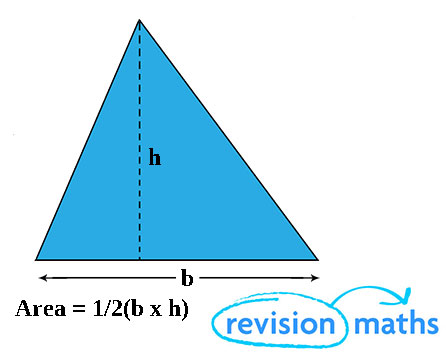½ b is half the base length of the triangle and h is the height of the triangle. So you multiply ½ the base length by the height.

Rectangle

To calculate the area of a rectangle you use the following formula: A = bh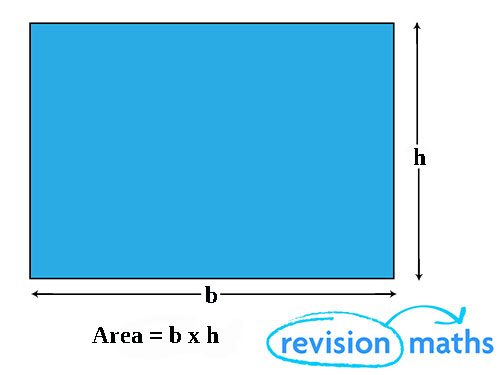This means you just multiply the base length by the height.

Parallelogram

The area of a parallelogram is calculated in exactly the same way as the area of a rectangle A = bh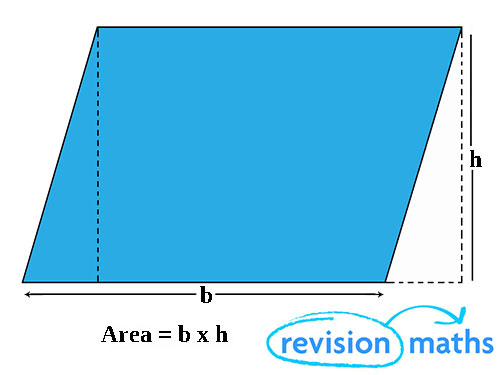Trapezium

The area of a trapezium can be calculated using the following formula: A = ½ (a + b)h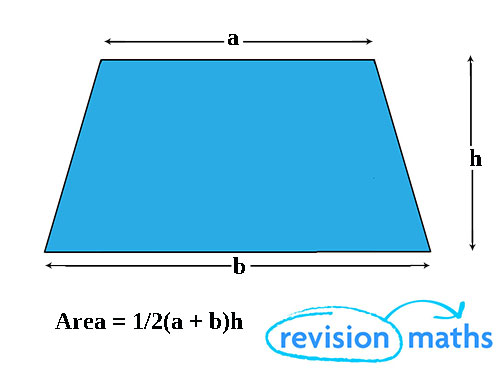So you add half the top length  (a) and half the base length (b) together and multiply these by the height (h).

Circle

The area of a circle is calculated using this formula: A =  πr2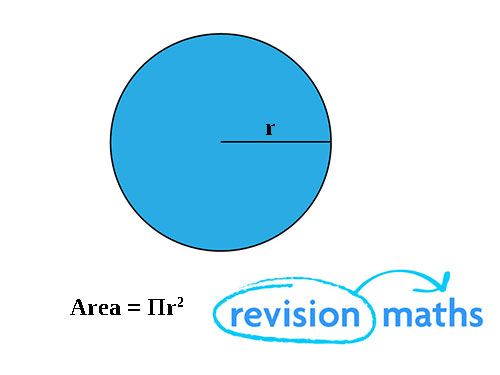π is the Greek letter pi (use the π symbol on your calculator) then times pi by the radius of the circle (r) squared.